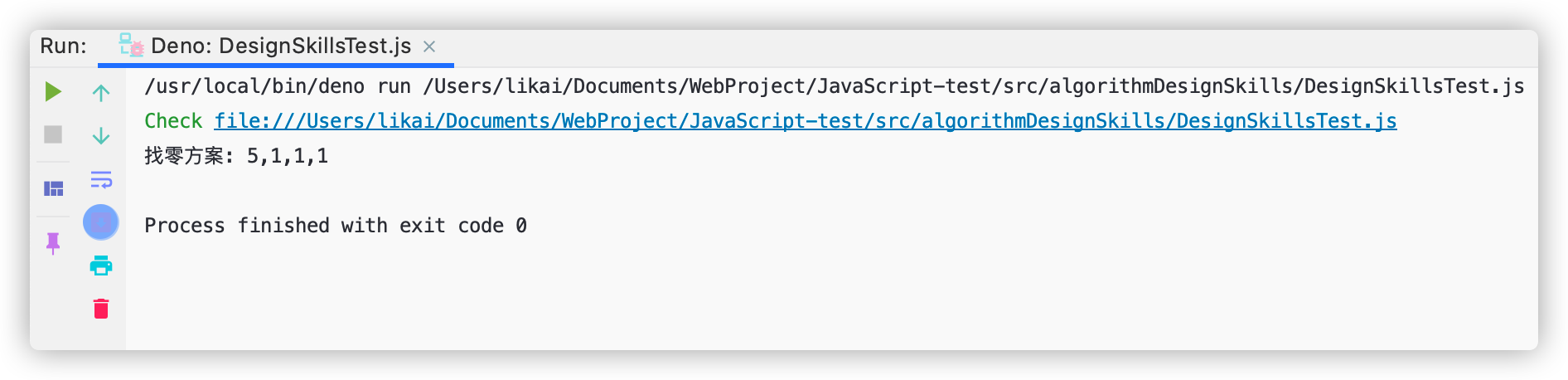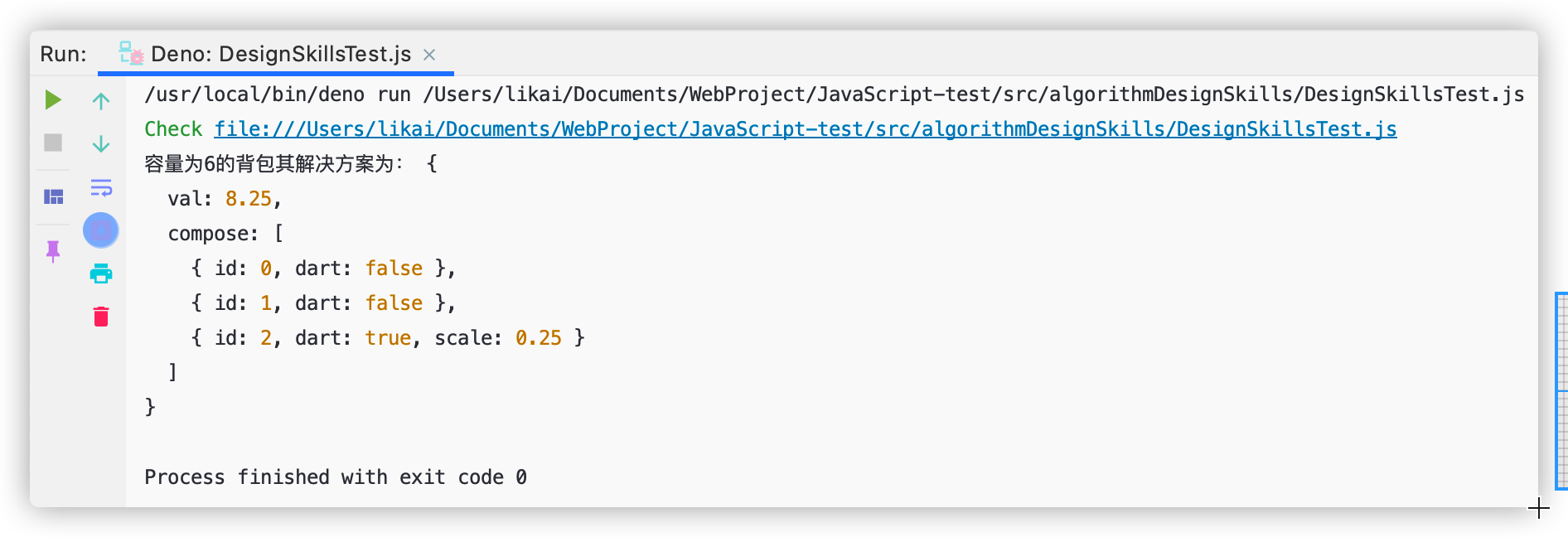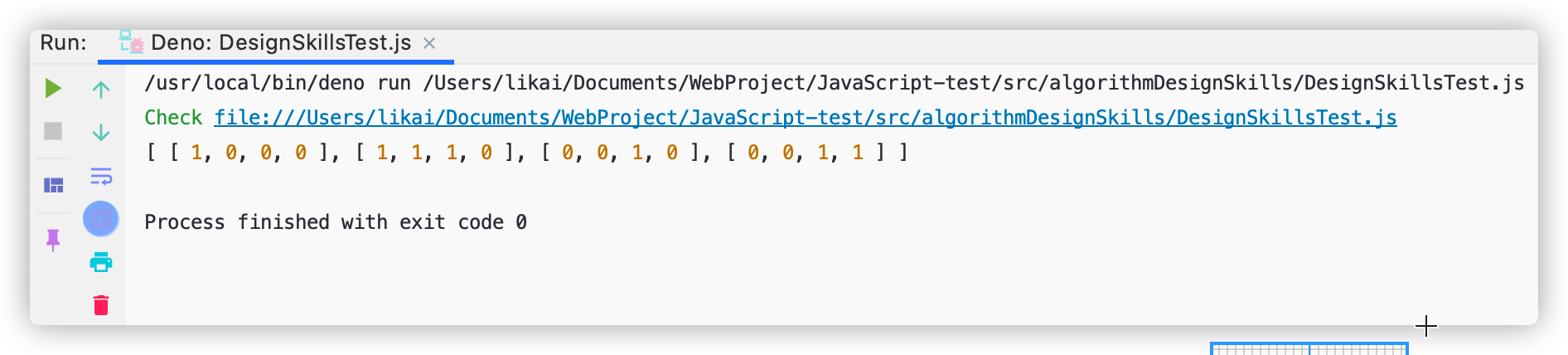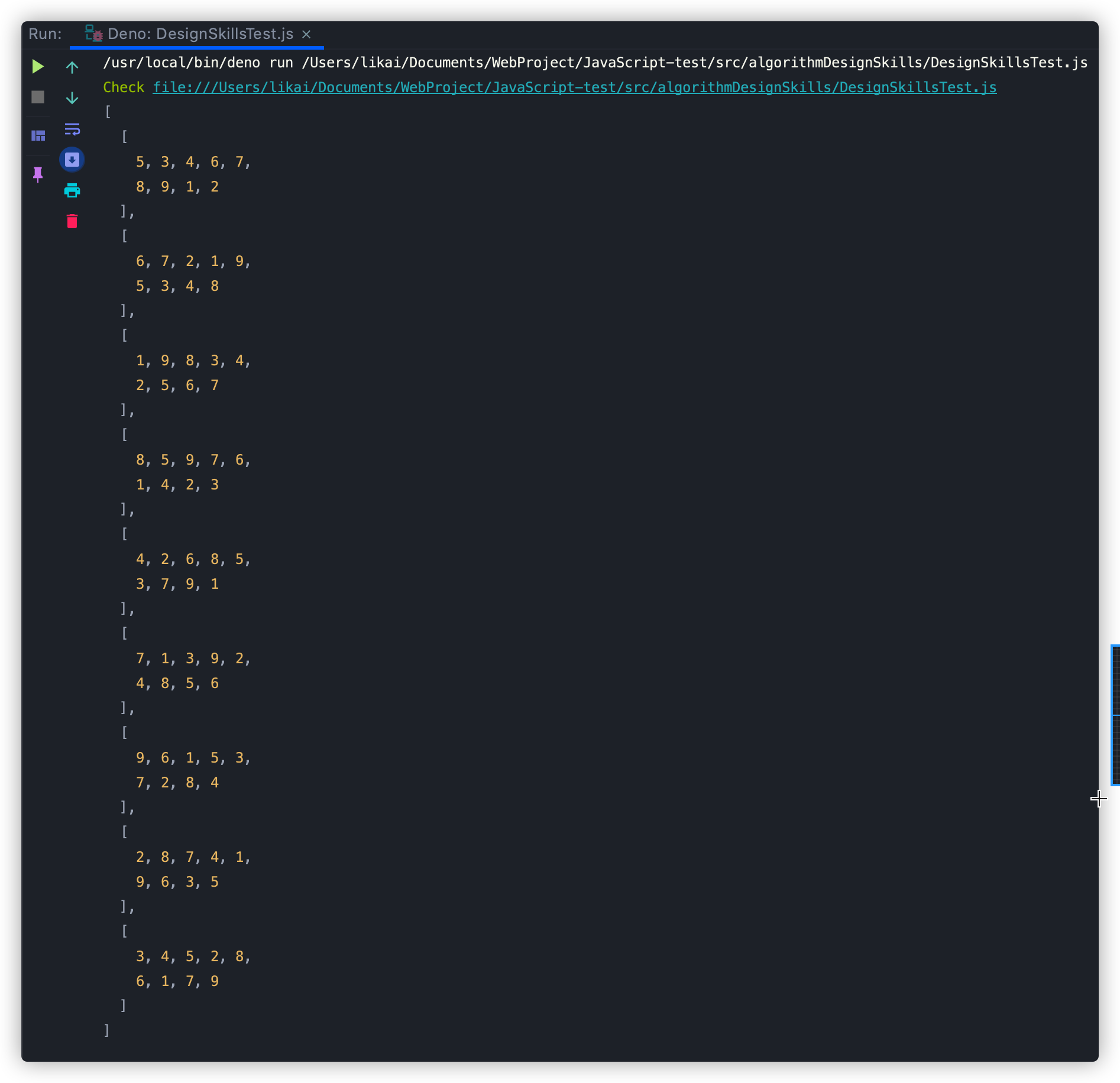• 累计撰写 164 篇文章
• 累计创建 25 个标签
• 累计收到 208 条评论

### 目 录CONTENT# 实现贪心算法与回溯算法2020-09-14 / 0 评论 / 3 点赞 / 336 阅读 / 8,371 字

## 贪心算法

### 实例讲解

#### 最少硬币找零问题

##### 实现思路
• 需要两个参数：硬币面额coins、找零金额amount
• 声明辅助变量change，用于存储找零方案
• 声明辅助变量total，用于存储当前已找零金额
• 从大到小遍历coins
• 取出当前遍历到的面额，判断当前取出的面额加上total，其值是否小于amount
• 如果小于等于，则执行while循环，将当前面额放入找零方案中，total的值加上当前面额
• 否则退出while循环，继续下一轮for循环，直至coins被取完
• 循环结束，找零方案已计算完毕，返回找零方案change
##### 实现代码

knapSackGreedy(capacity: number, weights: number[], values: number[]): number {
const n = values.length;
// 已装入背包的物品总重量
// 已装入背包的物品总价值
let val = 0;
for (let i = 0; i < n && load < capacity; i++) {
// 物品可以完整的放入背包
if (weights[i] <= capacity - load) {
// 将物品的价值计入背包已装入物品的总价值
val += values[i];
// 将物品的重量计入背包已装入物品的总重量
} else {
// 物品无法完整的放入背包，计算能够装入部分的比例
const r = (capacity - load) / weights[i];
// 将计算出的物品价值计入背包已装入物品的总价值
val += r * values[i];
// 将物品的重量计入背包已装入物品的总重量
}
}
// 返回物品总价值
return val;
}

##### 编写测试代码

const designSkills = new DesignSkills();
const result = designSkills.minCoinChangeGreedy([1, 5, 10, 25], 8);
console.log(找零方案: \${result});#### 背包问题

1 2 3
2 3 4
3 4 5

##### 实现思路

• 需要3个参数：背包容量capacity、物品重量weights、物品价值values
• 声明三个辅助变量：物品数量n、已装入背包的物品总重量load、已装入背包的物品总价值val
• 遍历背包中的物品，终止条件为当前遍历到的元素小于nload小于capacity
• 如果当前遍历到的物品重量weights[i]小于等于背包容量capacity - 以装入背包的物品总量load，则代表物品可以完整的放入背包，将当前物品的重量和价值计入已装入背包中
• 否则，物品无法完整放入背包，计算能够装入部分的比例，计算方法为：（背包容量-已装入背包的物品总重量）/ 当前要放入背包的物品重量
• 用计算出来的比例*当前物品的价值，将得出的结果计入以装入背包的物品总价值中。将当前物品的重量的计入已装入背包的总重量中。
• 遍历结束，物品价值计算完毕，返回已装入物品总价值。
##### 实现代码

/**
* 贪心算法: 背包问题
* @param capacity 背包容量
* @param weights 物品重量
* @param values 物品价值
*/
knapSackGreedy(
capacity: number,
weights: number[],
values: number[]
): { val: number; compose: ({ dart: boolean; scale: number; id: number } | { dart: boolean; id: number })[] } {
const n = values.length;
// 存储解决方案
const compose = [];
// 已装入背包的物品总重量
// 已装入背包的物品总价值
let val = 0;
for (let i = 0; i < n && load < capacity; i++) {
// 物品可以完整的放入背包
if (weights[i] <= capacity - load) {
// 将物品的价值计入背包已装入物品的总价值
val += values[i];
// 将物品的重量计入背包已装入物品的总重量
// 当前物品可以完整放入，将物品编号放入组合方案中
compose.push({ id: i, dart: false });
} else {
// 物品无法完整的放入背包，计算能够装入部分的比例
const r = (capacity - load) / weights[i];
// 将计算出的物品价值计入背包已装入物品的总价值
val += r * values[i];
// 将物品的重量计入背包已装入物品的总重量
// 当前物品无法完整放入，将物品编号和可放物品比例放入组合方案中
compose.push({ id: i, dart: true, scale: r });
}
}
// 返回物品总价值
return { val: val, compose: compose };
}

##### 编写测试代码

const designSkills = new DesignSkills();
const values = [3, 4, 5],
weights = [2, 3, 4],
capacity = 6;
console.log("容量为6的背包其解决方案为：", designSkills.knapSackGreedy(capacity, weights, values));## 回溯算法

### 实例讲解

#### 迷宫老鼠问题

1. 给定一个大小为N*N的矩阵，矩阵的每个位置都是一个方块。
2. 每个位置的值为0或1
3. 0表示这个格子有障碍物不能走，1表示这个格子为空闲状态可以走

S
D

M 0 1 2 3
0 1 0 0 0
1 1 1 1 1
2 0 0 1 0
3 0 1 1 1

 ->  ->  ->  -> ] ->  -> 

##### 实现思路

• 接收一个参数maze，其类型为一个二维数组，表示迷宫主体。
• 声明辅助变量solution，用于存放解决方案
• 初始化solution，将所有格子填充为o
• 从起始位置开始寻找路径，更新solution
• 寻找路径方法返回true则返回solution，否则返回无解

• 寻找路径函数接收4个参数：横纵坐标x, y、迷宫maze、解决方案solution
• 由于该函数为递归实现，因此我们先确立递归的基准条件：当x和y都到终点时。即：x = n-1 && y = n-1，满足条件时，我们将解决方案的最后一个位置标为1然后返回解决方案
• 判断迷宫x,y位置的值是否可走，判断条件为：x和y的值必须大于等于0且x和y的值必须必须小于迷宫的长度且x,y位置的值不为0
• 如果可以走，则将solution该格子的值改为1
• 随后，老鼠的位置向下移动一格，即x+1,用新的值递归调用寻找路径函数
• 向下移动的过程中，如果遇到格子的值为0时，则向右移动老鼠的位置，即y+1，用新的值递归调用寻找路径函数。
• 上述两个条件都无法满足，则表示老鼠水平和垂直都不能移动，则将该格子的值改为0，表示无法移动，回溯，即将当前层从递归栈中移除，寻找另一种解决方案。
• 当所有方案都尝试完毕后还是未能找到解，则代表该迷宫无解，返回false。

S 0 1 2 3
0 1 0 0 0
1 1 1 1 0
2 0 0 1 0
3 0 0 1 1
##### 实现代码

/**
*  回溯算法：迷宫老鼠问题
*
* @param maze 迷宫
*/
ratInAmaze(maze: number[][]): number[][] | string {
// 解决方案
const solution: number[][] = [];
for (let i = 0; i < maze.length; i++) {
solution[i] = [];
for (let j = 0; j < maze[i].length; j++) {
solution[i][j] = 0;
}
}
// 寻找路径
if (this.findPath(0, 0, maze, solution)) {
// 返回解决方案
return solution;
}
// 无解
return "此迷宫无解";
}
// 寻找路径
findPath(x: number, y: number, maze: number[][], solution: number[][]): boolean {
const n = maze.length;
// 递归基准条件：老鼠走到了迷宫的尽头
if (x === n - 1 && y === n - 1) {
// 将最后一个位置标记为路径的一部分
solution[x][y] = 1;
return true;
}
// 判断老鼠能否安全移动到该位置
if (this.isSafe(maze, x, y)) {
// 该位置可以移动，将其标注为可移动
solution[x][y] = 1;
// 沿着迷宫的行移动
if (this.findPath(x + 1, y, maze, solution)) {
return true;
}
// 沿着迷宫的列移动
if (this.findPath(x, y + 1, maze, solution)) {
return true;
}
// 水平和垂直都无法移动，将这步路径标注为不可移动
solution[x][y] = 0;
// 回溯，即将当前层从递归栈中移除，尝试另一种解决方案
return false;
}
// 所有移动方案都尝试完毕，都无法移动，则退出当前递归
return false;
}
// 验证此位置是否能走
isSafe(maze: number[][], x: number, y: number): boolean {
const n = maze.length;
// x和y必须大于等于0且迷宫的第x行y列不能为0老鼠就可以走
return x >= 0 && y >= 0 && x < n && y < n && maze[x][y] !== 0;
}

##### 编写测试代码
const designSkills = new DesignSkills();
// 迷宫老鼠问题
const RatResult = designSkills.ratInAmaze([
[1, 0, 0, 0],
[1, 1, 1, 1],
[0, 0, 1, 0],
[0, 1, 1, 1]
]);
console.log(RatResult);#### 数独解题器

• 由一个9*9的矩阵组成
• 矩阵的每行每列都由1~9这9个数字组成，且不重复
• 矩阵中还包含了3*3的小矩阵，同样由9个数字组成，且不重复。
• 游戏开始前会提供一个数独矩阵，它填充了部分数字，未填充部分用0表示

S 0 1 2 3 4 5 6 7 8
0 5 3 7
1 6 1 9 5
2 9 8 6
3 8 6 3
4 4 8 3 1
5 7 2 6
6 6 2 8
7 4 1 9 5
8 8 7 9

S 0 1 2 3 4 5 6 7 8
0 5 3 4 6 7 8 9 1 2
1 6 7 2 1 9 5 3 4 8
2 1 9 8 3 4 2 5 6 7
3 8 5 9 7 6 1 4 2 3
4 4 2 6 8 5 3 7 9 1
5 7 1 3 9 2 4 8 5 6
6 9 6 1 5 3 7 2 8 4
7 2 6 7 4 1 9 6 3 5
8 3 4 5 2 8 6 1 7 9
##### 实现思路

• 接收一个参数matrix,即数独。
• 调用递归函数，填充数独。
• 如果递归函数将数独填充完毕，则返回填充好的数独。否则返回错无解。

• 接收一个参数matrix，即待填充的数独
• 我们声明三个辅助变量row, col, checkBankSpaces分别用于描述数独的行、列、当前格子是否为空
• 遍历数独，寻找空格子，记录空格子的位置，即：row, col
• 递归基线条件：格子不为空
• 为空格子填充数字，判断其是否满足数独的填充规则
• 如果满足规则就往空格子填充对应的数字
• 继续递归，寻找空格子进行填充
• 所有数字都尝试完后，仍然不满足规则，就填充0
• 回溯，返回上一个递归栈

• 当前填充的数字在其行中不重复
• 当前填充的数字在其列中不重复
• 当前填充的数字在其3*3的矩阵中不重复
##### 实现代码

/**
* 数独解题器
* 游戏规则：
*        1. 用数字1~9填满一个9*9的矩阵
*        2. 矩阵的每行每列都由1～9这九个数字组成，且不能重复
*        3. 矩阵还包含了3*3的小矩阵，同样需要用这9个数字填满，填充时其值所在的小矩阵中不能有重复的数字
*        4. 游戏开始前会提供一个数独矩阵，它填了部分数字，未填充部分用0表示
* @param matrix 数独矩阵
*/
sudokuSolver(matrix: number[][]): number[][] | string {
if (this.solveSudoku(matrix)) {
return matrix;
}
return "此问题无解";
}
/**
* 解数独
* @param matrix 数独
* @private
*/
private solveSudoku(matrix: number[][]) {
// 辅助变量用于描述数独的行和列
let row: number;
let col = 0;
// 检查格子是否为空
let checkBlankSpaces = false;
// 寻找空格子
for (row = 0; row < matrix.length; row++) {
for (col = 0; col < matrix[row].length; col++) {
// 检测到空格子，终止内层循环
if (matrix[row][col] === this.UNASSIGNED) {
checkBlankSpaces = true;
break;
}
}
// 格子为空终止外层循环
if (checkBlankSpaces) {
break;
}
}
// 格子不为空时终止递归
if (!checkBlankSpaces) {
return true;
}
// 为空格子填充数字，判断其是否满足数独的填充规则
for (let num = 1; num <= 9; num++) {
// 如果满足规则，就往空格子填充num
if (DesignSkills.MatrixIsSafe(matrix, row, col, num)) {
matrix[row][col] = num;
// 递归，继续寻找空格子，然后填充
if (this.solveSudoku(matrix)) {
return true;
}
// 所有数字都尝试完后，仍然不满足则填充0
matrix[row][col] = this.UNASSIGNED;
}
}
// 回溯，即将返回到上一个递归栈
return false;
}
/**
* 校验当前值是否冲突
* @param matrix
* @param row
* @param col
* @param num
* @private
*/
private static MatrixIsSafe(matrix: number[][], row: number, col: number, num: number): boolean {
// 当num的值不再当前行，不在当前列，不在3*3的小格子中时则表示num不冲突
return (
!DesignSkills.usedInRow(matrix, row, num) &&
!DesignSkills.usedInCol(matrix, col, num) &&
!DesignSkills.usedInBox(matrix, row - (row % 3), col - (col % 3), num)
);
}
/**
* 检测当前值是否在矩阵的指定行中
* @param matrix
* @param row
* @param num
* @private
*/
private static usedInRow(matrix: number[][], row: number, num: number): boolean {
for (let col = 0; col < matrix.length; col++) {
if (matrix[row][col] === num) {
return true;
}
}
return false;
}
/**
* 检测当前值是否在矩阵的指定列中
* @param matrix
* @param col
* @param num
* @private
*/
private static usedInCol(matrix: number[][], col: number, num: number): boolean {
for (let row = 0; row < matrix.length; row++) {
if (matrix[row][col] === num) {
return true;
}
}
return false;
}
/**
* 检测当前值是否在3*3的小矩阵中
* @param matrix
* @param boxStartRow
* @param boxStartCol
* @param num
* @private
*/
private static usedInBox(matrix: number[][], boxStartRow: number, boxStartCol: number, num: number): boolean {
for (let row = 0; row < 3; row++) {
for (let col = 0; col < 3; col++) {
if (matrix[row + boxStartRow][col + boxStartCol] === num) {
return true;
}
}
}
return false;
}

##### 编写测试代码
const designSkills = new DesignSkills();
// 数独解题器
const sudokuGrid = [
[5, 3, 0, 0, 7, 0, 0, 0, 0],
[6, 0, 0, 1, 9, 5, 0, 0, 0],
[0, 9, 8, 0, 0, 0, 0, 6, 0],
[8, 0, 0, 0, 6, 0, 0, 0, 3],
[4, 0, 0, 8, 0, 3, 0, 0, 1],
[7, 0, 0, 0, 2, 0, 0, 0, 6],
[0, 6, 0, 0, 0, 0, 2, 8, 0],
[0, 0, 0, 4, 1, 9, 0, 0, 5],
[0, 0, 0, 0, 8, 0, 0, 7, 9]
];
console.log(designSkills.sudokuSolver(sudokuGrid));## 写在最后

• 文中如有错误，欢迎在评论区指正，如果这篇文章帮到了你，欢迎点赞和关注😊
• 本文首发于掘金，未经许可禁止转载💌
3